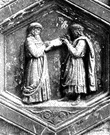# Pythagoras

(redirected from Pythagoreas)
Also found in: Encyclopedia.

## Py·thag·o·ras

(pĭ-thăg′ər-əs) fl. sixth century bc.
Greek philosopher who founded a school in southern Italy that sought to discover the mathematical principles of reality through the study of musical harmony and geometry. The Pythagorean theorem is ascribed to him.

## Pythagoras

(paɪˈθæɡərəs)
n
(Biography) ?580–?500 bc, Greek philosopher and mathematician. He founded a religious brotherhood, which followed a life of strict asceticism and greatly influenced the development of mathematics and its application to music and astronomy

## Pythagoras

(paɪˈθæɡərəs)
n
(Astronomy) a deep crater in the NE quadrant of the moon, 136 kilometres in diameter

## Py•thag•o•ras

(pɪˈθæg ər əs)

n.
c582–c500 B.C., Greek philosopher and mathematician.

## Py·thag·o·ras

(pĭ-thăg′ər-əs)
Sixth century b.c. Greek philosopher who theorized that numbers constitute the essence of all natural things. He developed the Pythagorean theorem and was one of the first to apply mathematical order to observations of the stars.
ThesaurusAntonymsRelated WordsSynonymsLegend:
 Noun 1Pythagoras - Greek philosopher and mathematician who proved the Pythagorean theorem; considered to be the first true mathematician (circa 580-500 BC)
Translations
Pythagoras
Pythagoras
Pythagoras
Pythagoras
Pythagoras
Pitagora
Pythagoras
Pythagoras
Pythagoras
Pythagoras
Pitagoras
Pitagora
Pitagora
Pythagoras

## Pythagoras

[paɪˈθægərəs] NPitágoras

nPythagoras m

## Pythagoras

[paɪˈθægərəs] n
Pythagoras' theorem →
Site: Follow: Share:
Open / Close